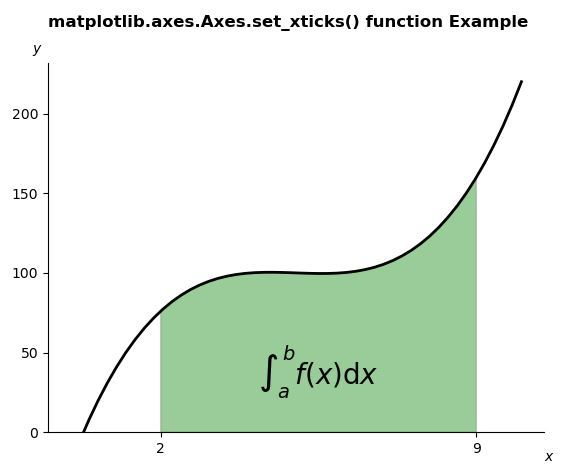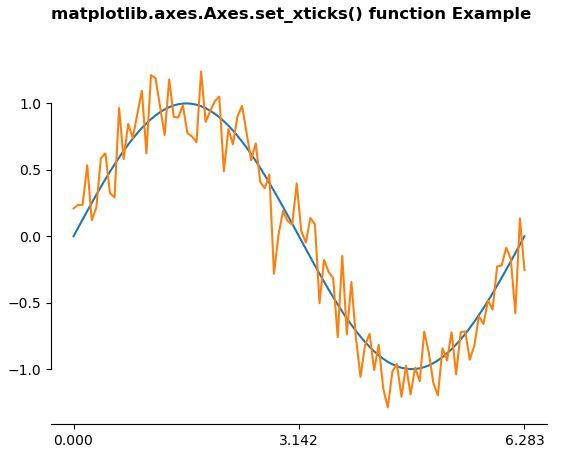# Matplotlib.axes.Axes.set_xticks() in Python

Matplotlib is a library in Python and it is numerical – mathematical extension for NumPy library. The Axes Class contains most of the figure elements: Axis, Tick, Line2D, Text, Polygon, etc., and sets the coordinate system. And the instances of Axes supports callbacks through a callbacks attribute.

## matplotlib.axes.Axes.set_xticks() Function

The Axes.set_xticks() function in axes module of matplotlib library is used to Set the x ticks with list of ticks.

Syntax: Axes.set_xticks(self, ticks, minor=False)

Parameters: This method accepts the following parameters.

• ticks : This parameter is the list of x-axis tick locations.
• minor : This parameter is used whether set major ticks or to set minor ticks

Return value: This method does not returns any value.

Below examples illustrate the matplotlib.axes.Axes.set_xticks() function in matplotlib.axes:

Example 1:

 # Implementation of matplotlib function  import numpy as np  import matplotlib.pyplot as plt  from matplotlib.patches import Polygon          def func(x):      return (x - 4) * (x - 6) * (x - 5) + 100         a, b = 2, 9  # integral limits  x = np.linspace(0, 10)  y = func(x)      fig, ax = plt.subplots()  ax.plot(x, y, "k", linewidth = 2)  ax.set_ylim(bottom = 0)      # Make the shaded region  ix = np.linspace(a, b)  iy = func(ix)  verts = [(a, 0), *zip(ix, iy), (b, 0)]  poly = Polygon(verts, facecolor ='green',                 edgecolor ='0.5', alpha = 0.4)  ax.add_patch(poly)      ax.text(0.5 * (a + b), 30,          r"$\int_a ^ b f(x)\mathrm{d}x$",          horizontalalignment ='center',          fontsize = 20)      fig.text(0.9, 0.05, '$x$')  fig.text(0.1, 0.9, '$y$')      ax.spines['right'].set_visible(False)  ax.spines['top'].set_visible(False)   ax.set_xticks((a, b))      fig.suptitle('matplotlib.axes.Axes.set_xticks()\   function Example\n\n', fontweight ="bold")  fig.canvas.draw()  plt.show()

Output:Example 2:

 # Implementation of matplotlib function  import numpy as np  import matplotlib.pyplot as plt      # Fixing random state for reproducibility  np.random.seed(19680801)      x = np.linspace(0, 2 * np.pi, 100)  y = np.sin(x)  y2 = y + 0.2 * np.random.normal(size = x.shape)      fig, ax = plt.subplots()  ax.plot(x, y)  ax.plot(x, y2)     ax.set_xticks([0, np.pi, 2 * np.pi])      ax.spines['left'].set_bounds(-1, 1)  ax.spines['right'].set_visible(False)  ax.spines['top'].set_visible(False)      fig.suptitle('matplotlib.axes.Axes.set_xticks() \  function Example\n\n', fontweight ="bold")  fig.canvas.draw()  plt.show()

Output:Attention geek! Strengthen your foundations with the Python Programming Foundation Course and learn the basics.

To begin with, your interview preparations Enhance your Data Structures concepts with the Python DS Course.

My Personal Notes arrow_drop_upSmall things always make you to think big

If you like GeeksforGeeks and would like to contribute, you can also write an article using contribute.geeksforgeeks.org or mail your article to contribute@geeksforgeeks.org. See your article appearing on the GeeksforGeeks main page and help other Geeks.

Please Improve this article if you find anything incorrect by clicking on the "Improve Article" button below.

Article Tags :

Be the First to upvote.

Please write to us at contribute@geeksforgeeks.org to report any issue with the above content.